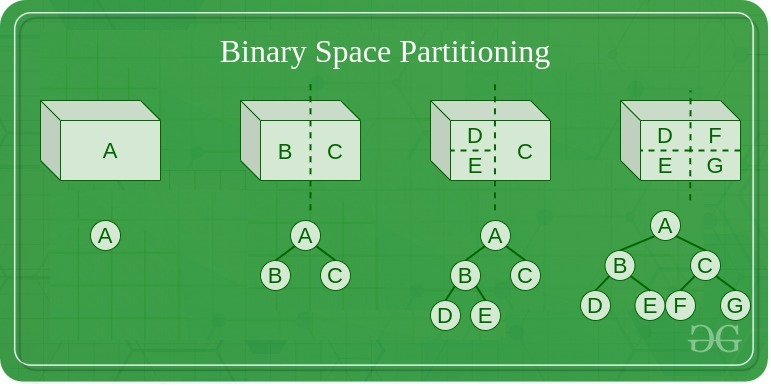# Binary Space Partitioning

• Difficulty Level : Hard
• Last Updated : 30 Sep, 2020

Binary Space Partitioning is implemented for recursively subdividing a space into two convex sets by using hyperplanes as partitions. This process of subdividing gives rise to the representation of objects within the space in the form of tree data structure known as BSP Tree.
Binary space partitioning arose in the context of 3D computer graphics in 1969, where the structure of a BSP tree allows for spatial information about the objects in a scene that is useful in rendering, such as objects being ordered from front-to-back with respect to a viewer at a given location, to be accessed rapidly.### Needs of Binary Space Partitioning

Binary space partitioning arose from the computer graphics need to rapidly draw three-dimensional scenes composed of polygons. A simple way to draw such scenes is the painter’s algorithm, which produces polygons in order of distance from the viewer, back to front, painting over the background, and previous polygons with each closer object. This approach has two disadvantages: the time required to sort polygons in back to front order, and the possibility of errors in overlapping polygons. Fuchs and co-authors showed that constructing a BSP tree solved both of these problems by providing a rapid method of sorting polygons with respect to a given viewpoint (linear in the number of polygons in the scene) and by subdividing overlapping polygons to avoid errors that can occur with the painter’s algorithm.

### Overview of BSP

Binary space partitioning is treated as a generic process of recursively dividing a scene into two until the partitioning satisfies one or more requirements. It can be viewed as a generalization of other spatial tree structures such as k-d trees and quadtrees, one where hyperplanes that partition space may have any orientation instead of being aligned with the coordinate axes as they are in k-d trees or quadtree.
When implemented in computer graphics to render scenes composed of planar polygons, the partitioning planes are frequently selected to coincide with the planes defined by polygons in the scene. The specific choice of partitioning plane and criterion for completing the partitioning process varies depending on the purpose of the BSP tree.
For example: In computer graphics rendering, the scene is divided until and unless each node of the BSP tree consists of only polygons that can render in arbitrary order. When back-face culling is implemented, each node, therefore, consists of a convex set of polygons, whereas when rendering double-sided polygons, each node of the BSP tree consists of only polygons in a single plane.

### Algorithm of generating a BSP Tree from a list of polygons

• Select a polygon P from the list.
• Make a node N in the BSP tree, and add P to the list of polygons at that node.
• For each other polygon in the list:
• If that polygon is wholly in front of the plane containing P, move that polygon to the list of nodes in front of P.
• If that polygon is wholly behind the plane containing P, move that polygon to the list of nodes behind P.
• If that polygon is intersected by the plane containing P, split it into two polygons and move them to the respective lists of polygons behind and in front of P.
• If that polygon lies in the plane containing P, add it to the list of polygons at node N.
• Apply this algorithm to the list of polygons in front of P.
• Apply this algorithm to the list of polygons behind P.

### Disadvantage of BSP

• Generating a BSP tree can be time-consuming.
• BSP does not solve the problem of visible surface determination.

### Uses of BSP

• It is used in collision detection in 3D video games and robotics.
• It is used in ray tracing
• It is involved in the handling of complex spatial scenes.
My Personal Notes arrow_drop_up